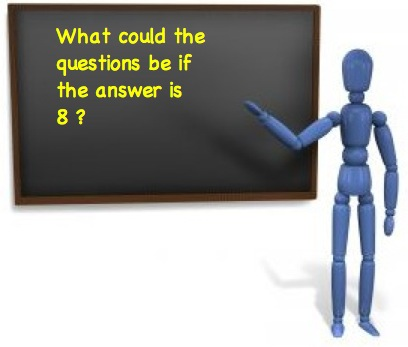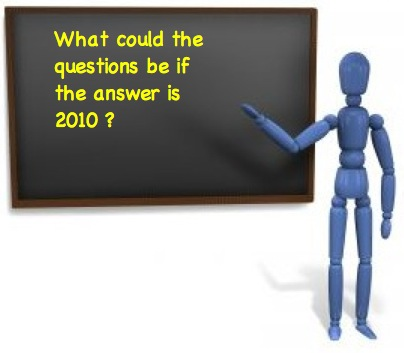#### You may also like### Prompt Cards

These two group activities use mathematical reasoning - one is numerical, one geometric.### Consecutive Numbers

An investigation involving adding and subtracting sets of consecutive numbers. Lots to find out, lots to explore.### Exploring Wild & Wonderful Number Patterns

EWWNP means Exploring Wild and Wonderful Number Patterns Created by Yourself! Investigate what happens if we create number patterns using some simple rules.

# It Was 2010!

##### Age 5 to 11Challenge Level

At the start of a new year teachers often like to use the number of that year.

Well I know that some teachers often use an idea like,The pupils would get things like:

$16 \div 2$
$20 - 4 - 4 - 4$
$6 + 2$
$\sqrt{64}$
----------

So here's a challenge to make an answer of $2010$You could try things like:
$$2000 + 10 \quad\quad 201 \times 10\quad\quad 500 + 1000 + 500 + 5 + 5$$

even this

$$19^2 +25^2 + 32^2$$

Don't forget you might like to use calculators or computers!

See what different questions you can find and compare yours with others.
Perhaps you can make up one that is easy, one that is harder and one that is very hard.

Now that $2010$ has passed try the same for the year we have now!